# Fourbar2

One kinematic loop with four bars (with UniversalSpherical joint; 1 non-linear equation)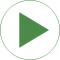# Information

This information is part of the Modelica Standard Library maintained by the Modelica Association.

This is a second version of the "four-bar" mechanism, see figure: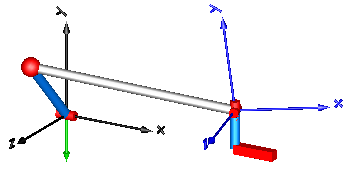In this case the three revolute joints on the left top-side and the two revolute joints on the right top side have been replaced by the joint UniversalSpherical that is a rod connecting a spherical and a universal joint. This joint is defined by 1 constraint stating that the distance between the two spherical joints is constant. Using this joint in a kinematic loop reduces the sizes of non-linear algebraic equations. For this loop, only one non-linear algebraic system of equations of order 1 remains.

At the UniversalSpherical joint an additional frame_ia fixed to the rod is present where components can be attached to the connecting rod. In this example just a coordinate system is attached to visualize frame_ia (coordinate system on the right in blue color).

Another feature is that the length of the connecting rod can be automatically calculated during initialization. In order to do this, another initialization condition has to be given. In this example, the initial value of the distance of the prismatic joint j2 has been fixed (via the "Initialization" menu) and the rod length of joint "UniversalSpherical" is computed during initialization since parameter computeLength = true is set in the joint parameter menu. The main advantage is that during initialization no non-linear system of equation is solved and therefore initialization always works. To be precise, the following trivial non-linear equation is actually solved for rodLength:

```   rodLength*rodLength = f(angle of revolute joint, distance of prismatic joint)
```

# Outputs (4)

j1_phi Type: Angle (rad) Description: angle of revolute joint j1 Type: Position (m) Description: distance of prismatic joint j2 Type: AngularVelocity (rad/s) Description: axis speed of revolute joint j1 Type: Velocity (m/s) Description: axis velocity of prismatic joint j2

# Components (8)

world j1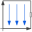Type: World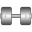Type: Revolute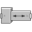Type: Prismatic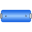Type: BodyCylinderType: BodyCylinder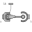Type: UniversalSpherical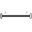Type: FixedTranslation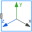Type: FixedFrame